# IoT Pressure Sensor: MKR GSM + Arduino Cloud + Google Sheets

IoT device to remotely monitor pressure (0-150 psi) using cellular data. Get notifications for pressures above/below high/low set-points.

IntermediateFull instructions provided9,160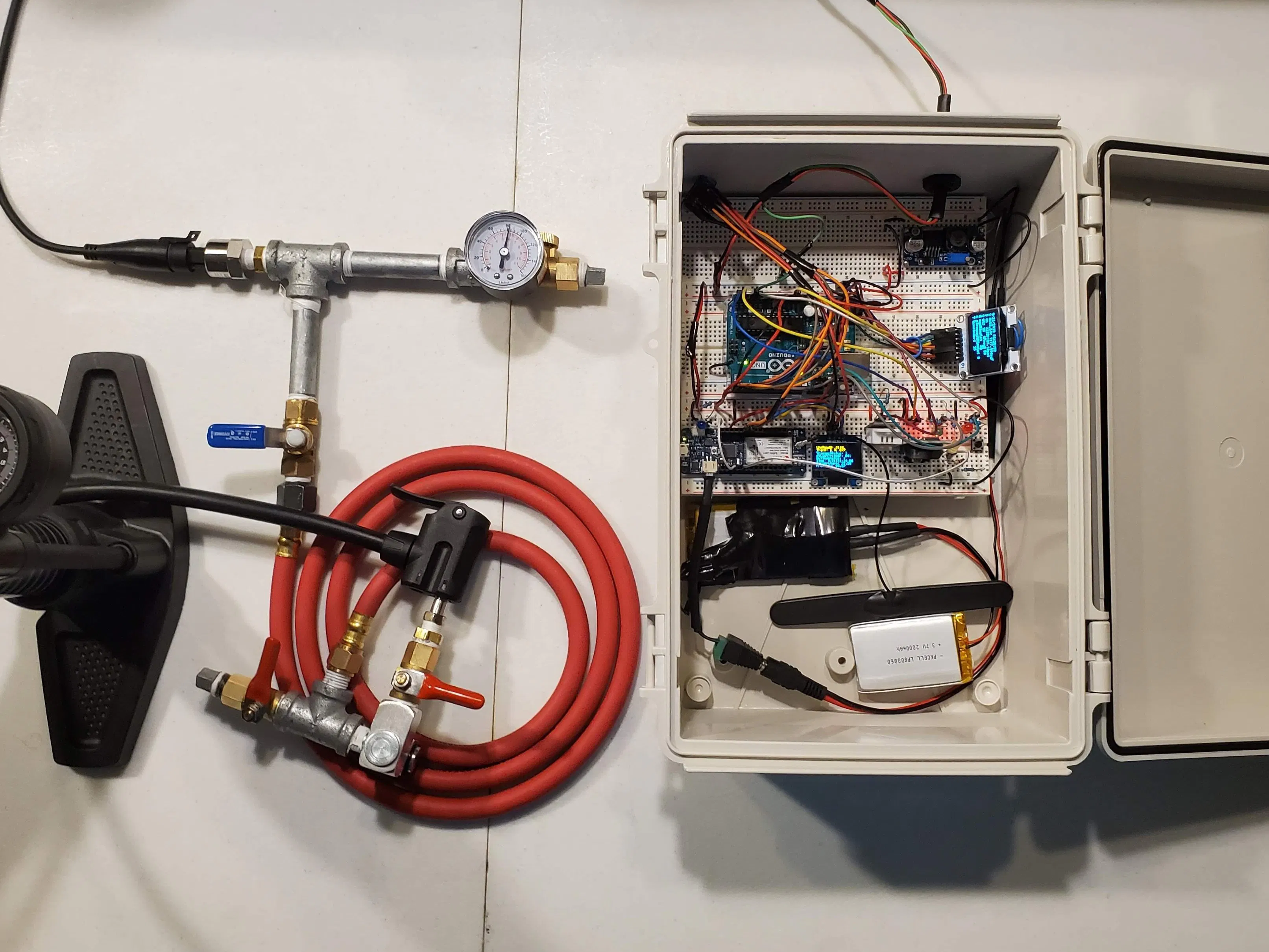## Things used in this project

### Hardware componentsArduino UNO & Genuino UNO
×1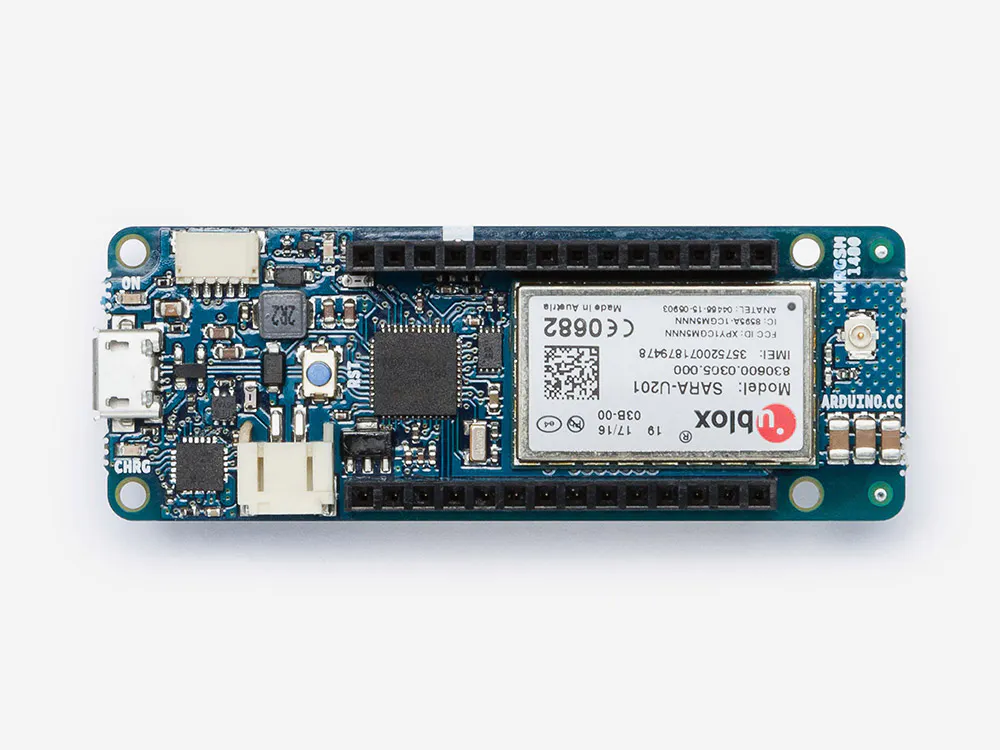Arduino MKR GSM 1400
×1
 Pressure Transducer (0-150 psi)
×1
 Adafruit DHT22 Temperature & Humidity Sensor
×1
 DS3231 RTC Module
×1
 Adafruit Logic Level Converter - BSS138 - 4-channel I2C-safe Bi-directional
×1
 Adafruit 3.7V LiPo Battery (2000 mAh or greater)
×2
 Voltage Step-up Converter
×1
 Basic Starter Kit with Assorted LEDs & Resistors
×1×1

### Software apps and online services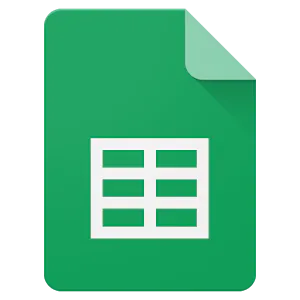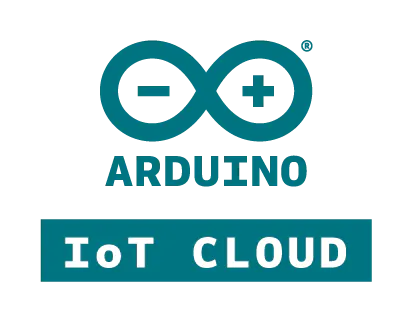Arduino IoT Cloud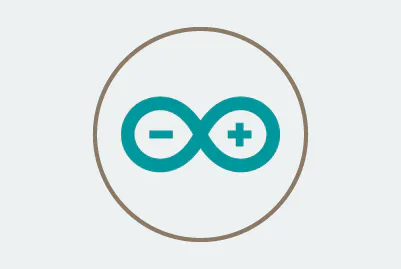Arduino Web EditorArduino IDE

## Schematics

### Schematic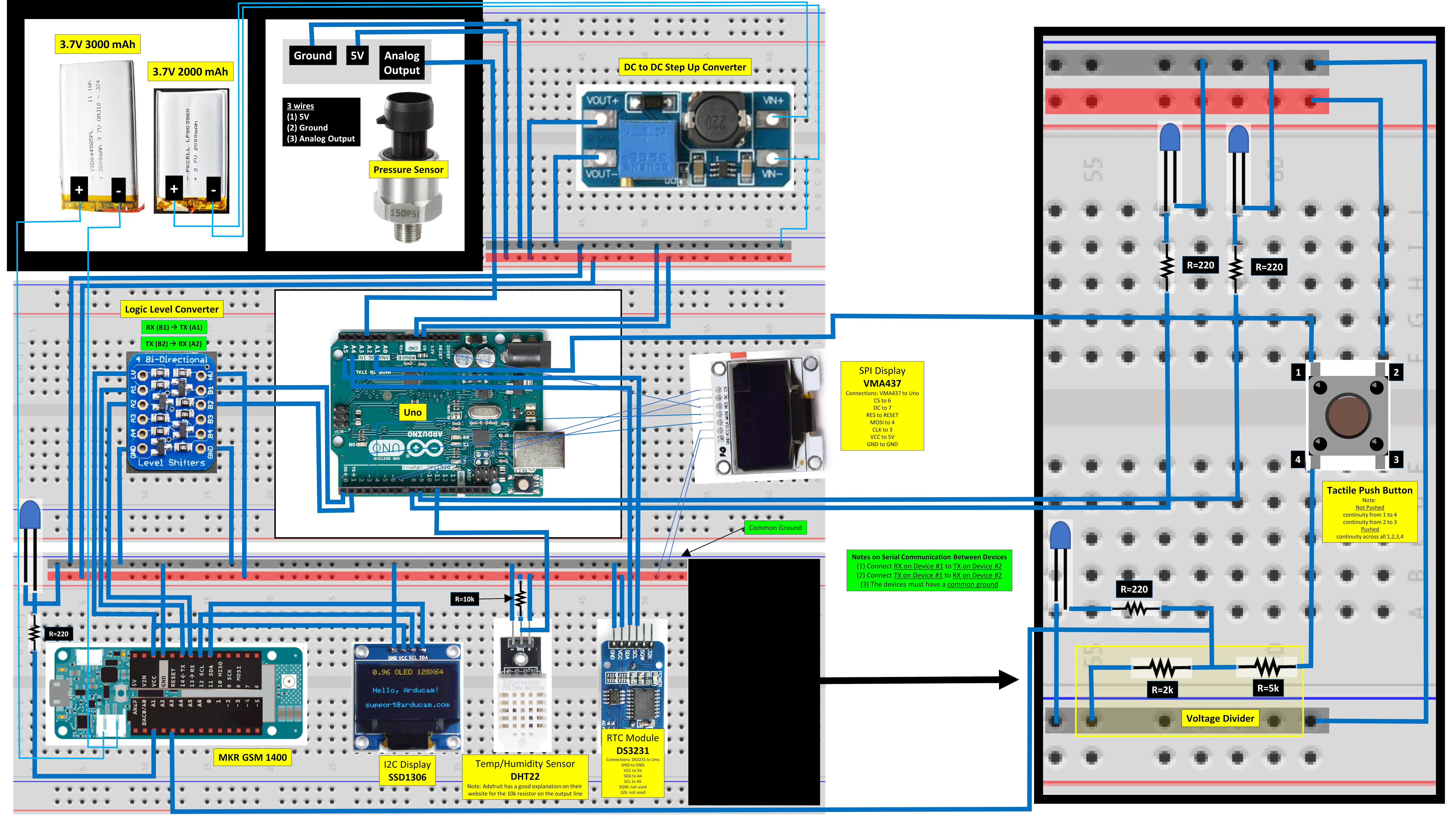## Code

### InstrumentReader - Sketch for Arduino Uno

Arduino
```// Sketch 1 of 2
// Arduino Uno
// Data is collected by this device and transmitted to the MKR 1400 via serial

// The DHT22 Sensor Requires 2 libraries, but only one is called in the code.
//     (1): DHT Sensor Library: https://github.com/adafruit/DHT-sensor-library

#include <SPI.h>
#include <Wire.h>
#include <DS3231.h>   // RTC module
#include <DHT.h>      // DHT22
#include "U8glib.h"   // Velleman 128 x 64 OLED SPI Display
// Note: Another standard U8glib library did not work when I attempted to use it for this display. It did work when I used the library recommended by the manufacturer.
// Syntax: https://github.com/olikraus/u8glib/wiki/userreference & https://github.com/olikraus/u8glib/wiki/thelloworld
//#include <SD.h>     // Option to save to SD card in Ethernet Shield for Arduino Uno

RTClib RTC;

#define DHTPIN 11     // Digital pin connected to the DHT sensor
#define DHTTYPE DHT22   // DHT 22  (AM2302), AM2321
DHT dht(DHTPIN, DHTTYPE);

U8GLIB_SH1106_128X64 u8g(3, 4, 6, 7); // (CLK/SCK: 3, MOSI: 4, CS: 6, DC(A0): 7) // u8g(sck, mosi, cs, a0 [, reset])

int y_pos = 0;  // global variable

//const int chipSelect = 10; // Option to save to SD card in Ethernet Shield for Arduino Uno
//float fileSizeSD = 0.0;    // Option to save to SD card in Ethernet Shield for Arduino Uno

int i = 0; // Count # of readings taken by the Uno (same as # loops in program)

const int ledPin = 9;     // transmit indicator (flashes when a data transmission occurs)
const int ledPin2 = 8;    // push transmit indicator (pushed high by manual button on breadboard or output from MKR 1400 activated from the cloud)
const int buttonPin = A1;

int buttonState = 0;

int transmitFrequency = 30;  // Frequency of Serial Print dataString for sending data to the second device (minutes)
String pTransmitDateTime = "";
int transmitCounter = 0;
int pTransmitMinute = 0;

float cRuntimeAtTriggerStart = 0.0;
float dtLastTrigger = 0.0;
int triggerCounter = 0.0;

// Input Variables to Control Trigger
float lowTrigger = 20.0;
float highTrigger = 40.0;
float dtLastTriggerLimit = 2.0; // If the condition is met for this length of time, an alert will be generated

void setup (void) {
Serial.begin(9600);
Wire.begin();
dht.begin();
pinMode(ledPin, OUTPUT);
pinMode(ledPin2, OUTPUT);
pinMode(buttonPin, INPUT);
u8g.setRot180();  // flip screen, if required (add/remove comments on this line to rotate)

// Option to save to SD card in Ethernet Shield for Arduino Uno
//    Serial.print("Initializing SD card...");
//    if (!SD.begin(chipSelect))     // see if the card is present and can be initialized
//    {
//      Serial.println("Card failed, or not present");
//      while (1);     // don't do anything more
//    }
//    Serial.println("card initialized.");
}

void loop (void) {
delay(5000);
DateTime now = RTC.now();
float cRuntime = millis()/60000;
float p = getPressure();
//    Serial.println(p);

//    Serial.print("Button: ");
//    Serial.println(buttonState);
if(buttonState == 1)
{
digitalWrite(ledPin2, HIGH);
delay(30000); // delay to allow MKR1400 to get ready to recieve data if Uno:buttonPin is pushed HIGH by MKR1400:pingPin
}
else
{
digitalWrite(ledPin2, LOW);
}

float t = dht.readTemperature(true);  // t = dht.readTemperature(true) --> temp if degrees F & t = dht.readTemperature() --> temp if degrees C

int transmitIndicator = 0;
if(now.minute() % transmitFrequency == 0 && now.minute() != pTransmitMinute)
{
transmitIndicator = 1;
pTransmitMinute = now.minute();
pTransmitDateTime = String(now.hour())+String(":")+String(now.minute())+String(":")+String(now.second());
}

int triggerStatus = 0;
if(p <= lowTrigger || p >= highTrigger)
{
// Note: the variable referenced in the condition for this if statement is evaulated against high & low setpoints
//    It is quick to change which variable is evaluated - this is the only location where the variable is specified

triggerStatus = 1;
triggerCounter++;
}
else
{
triggerCounter = 0;
}

if(triggerStatus == 1 && triggerCounter == 1)
{
cRuntimeAtTriggerStart = cRuntime;
}

dtLastTrigger = cRuntime - cRuntimeAtTriggerStart;

if((dtLastTrigger > dtLastTriggerLimit) && triggerStatus == 1)
{
}
else
{
}

{
flashLED(2,500);
}

// if(TriggerStatus existed for min specified time for Alert & Count = 1 meaining that this is the first loop where the time exceeded the min specified time
//       OR the triggerAlert status changes -- this will generate a push Alert if the TriggerStatus goes back to 0 meaning the Trigger conditions are no longer met.)
{
flashLED(5,500);
delay(5000);
}

ptriggerTransmitAlertIndicator = triggerTransmitAlertIndicator; // current indicator stored to previous indicator. On the next loop the value transferred here will be compared to the value generated based on new values.

// Create strings
String dataString = "";
String cDateTime = "";
String cHumTemp = "";
String cP = "";

dataString += "<"+String(i)+","+String(triggerTransmitAlertIndicator)+","+String(dtLastTrigger,0)+","+String(buttonState)+", "+String(now.month())+","+String(now.day())+","+String(now.year())+", "+String(now.hour())+","+String(now.minute())+","+String(now.second())+", "+String(h)+","+String(t)+","+String(p)+">";
cDateTime += String(now.month())+"/"+String(now.day())+"/"+String(now.year())+" "+String(now.hour())+":"+String(now.minute())+":"+String(now.second());
cHumTemp += "H:"+String(h)+"% T:"+String(t)+"degF";
cP += "P:"+String(p)+"psi";

if(transmitIndicator == 1 || triggerPushTransmitAlertIndicator == 1 || buttonState == 1)
{
char dataArray;
dataString.toCharArray(dataArray, 100);
Serial.println(dataArray);
flashLED(10,500);
transmitCounter++;
}

//    Serial.print("T:");
//    Serial.println(triggerStatus);

delay(100);     // wait a bit for the entire message to arrive
// picture loop
u8g.firstPage();
do
{
draw(cDateTime,cHumTemp, cP,i,transmitCounter,now.minute(),transmitFrequency,pTransmitMinute);
}
while(u8g.nextPage());
delay(1000);
// writeToSD(dataString);  // Option to save to SD card in Ethernet Shield for Arduino Uno
i++;
}

void draw(String DcDateTime,String DcHumTemp, String DcP, int Di, int DtransmitCounter,int DnowMinute,int DtransmitFrequency, int DpTransmitMinute)
{
u8g.begin();
u8g.setFont(u8g_font_5x7);  //u8g_font_micro //u8g_font_5x7  //u8g_font_5x8  //u8g_font_6x10
u8g.setFontPosTop();
u8g.setPrintPos(0,0);
u8g.print(DcDateTime);
u8g.setPrintPos(0,8);
u8g.print(2);
u8g.setPrintPos(10,8);
u8g.print(DcHumTemp);
u8g.setPrintPos(0,16);
u8g.print("3 #:");
u8g.setPrintPos(30,16);
u8g.print(Di);
u8g.setPrintPos(50,16);
u8g.print(DcP);
u8g.setPrintPos(0,24);
u8g.print("4 #t:");
u8g.setPrintPos(30,24);
u8g.print(DtransmitCounter);
u8g.setPrintPos(50,24);
u8g.print("tFreq: ");
u8g.setPrintPos(83,24);
u8g.print(DtransmitFrequency);
u8g.setPrintPos(0,32);
u8g.print(5);
u8g.setPrintPos(10,32);
u8g.print("nowMinute:");
u8g.setPrintPos(70,32);
u8g.print(DnowMinute);
u8g.setPrintPos(0,40);
u8g.print(6);
u8g.setPrintPos(10,40);
u8g.print("pTransmitMinute:");
u8g.setPrintPos(95,40);
u8g.print(DpTransmitMinute);
u8g.setPrintPos(0,48);
u8g.print(7);
u8g.setPrintPos(10,48);
u8g.print("Remainder:");
u8g.setPrintPos(70,48);
u8g.print(DnowMinute % DtransmitFrequency);
}

float getPressure()
{
//    Serial.print("Sensor Value: ");
//    Serial.print(sensorVal);
float voltage = (sensorVal*5.0)/1023.0;
//    Serial.print(" Volts: ");
//    Serial.print(voltage);
float m = ((150-0)/(4.5-0.4889));
float b = 150 - (m*4.5);
//    Serial.print(" m = ");
//    Serial.print(m);
//    Serial.print(" b = ");
//    Serial.print(b);
float pressure_psi = ((m*voltage)+ b);
//    Serial.print(" Pressure = ");
//    Serial.print(pressure_psi);
//    Serial.println(" psi");
//    delay(200);
return pressure_psi;
}

void flashLED(int num, int t)
{
for (int z = 1; z <= num; z++)
{
digitalWrite(ledPin, HIGH);
delay(t);
digitalWrite(ledPin, LOW);
delay(t);
}
}

// Option to save to SD card in Ethernet Shield for Arduino Uno
//void writeToSD(String dataToWrite)
//{
//    // open the file. note that only one file can be open at a time,
//    // so you have to close this one before opening another.
//    File dataFile = SD.open("datalog4.txt", FILE_WRITE);
//    fileSizeSD = dataFile.size();  // Reurns file size in bytes
//    fileSizeSD = fileSizeSD / 1000000 ;  // Converts bytes to MB. 1 MB = 1e6 bytes
//    // if the file is available, write to it:
//    if (dataFile)
//    {
//      dataFile.println(dataToWrite);
//      dataFile.close();
//      // print to the serial port too:
//      // Serial.println(dataToWrite);
//    }
//    // if the file isn't open, pop up an error:
//    else
//    {
//      Serial.println("error opening datalog1.txt");
//    }
//}
```

JavaScript
```// There are 2 functions in this code
// doPost function - Transmits data from the Arduino Cloud Webhook. It runs when there is new data on the Arduino Cloud.
// sendEmail function - Sends emails based on values extracted from the sheet named "Data" in the Google Sheets file for the project. It runs once every minute based on the settings in the trigger setup. For instructions, refer to the sendEmail function portion of the project hub post.

// The majority of this code (aside from the sendEmail function) is modeled after the following project on the Arduino Project Hub

// This a link to a GitHub repository with the Google Script used for the above mentioned project on the Project Hub.
// This link was copied from the desription of the project on the Project Hub.

// get active spreasheet

// get sheet named RawData
var sheet = ss.getSheetByName("RawData");
var sd = ss.getSheetByName("Data");
var sref =  ss.getSheetByName("References");

var MAX_ROWS = 1440;     // max number of data rows to display
// 3600s / cloud_int(30s) * num_ore(12h) = (60*60*12)/30 = (3600*12)/30 = 1440 readings in 12 hours at 30 second update interval
// (60*24)/15 = 96 readings in a 24 hour period at 15 minute update interval
var TIMESTAMP_COL = 1;  // column index of the timestamp column

function doPost(e) {
var cloudData = JSON.parse(e.postData.contents); // this is a json object containing all info coming from IoT Cloud
console.log(cloudData);
//var webhook_id = cloudData.webhook_id; // really not using these three
//var device_id = cloudData.device_id;
//var thing_id = cloudData.thing_id;
var values = cloudData.values; // this is an array of json objects
console.log(values);

// Store names and values from the values array
// Each incoming property has a:
//      name which will become the column names
//      value which will be written into the rows below the column header
var incLength = values.length;
var incNames = [];
var incValues = [];
for (var i = 0; i < incLength; i++) {
incNames[i] = values[i].name;
incValues[i] = values[i].value;
}

console.log(incNames);
console.log(incValues);

// read timestamp of incoming message
var timestamp = values.updated_at;          // format: yyyy-MM-ddTHH:mm:ss.mmmZ
var date = new Date(Date.parse(timestamp));

/*
This if statement is due to the fact that duplicate messages arrive from the cloud!
If that occurs, the timestamp is not read correctly and date variable gets compromised.
Hence, execute the rest of the script if the year of the date is well defined and it is greater
then 2018 (or any other year before)
*/
if (date.getYear() > 2018) {

// discard all messages that arrive 'late'
if (sheet.getRange(HEADER_ROW+1, 1).getValue() != '') {
// HEADER_ROW + 1 = Row #2 & Column #1 --> this is the location of the most recent timestamp in the sheet
// If the most recent timestamp is not blank = (''), then compare the current time to the timestamp of the incoming data
// If the most recent timestamp is blank, it is most likely the first time the script is run.
//      In this case skip this if statement and proceed to write in the column headers and data.
//      It doesnt matter if the data is arriving late (current time vs timestamp of incoming data).
var now = new Date(); // now
var COMM_TIME = 120; // Note: changed to 120 to allow more messages to come through, previously set at 5 seconds & worked ok // rough overestimate of communication time between cloud and app
if (now.getTime() - date.getTime() > COMM_TIME * 1000) {
// If the difference between the current time and the timestamp is greater than 5 seconds, discard the data.
// When the condition in this If statement evaluates true, the funtion will stop due to the return statement.
return; // "The return statement stops the execution of a function and returns a value from that function."
}
}

// This section writes values to the header row based on the names of the incoming properties
//      In other words, this section creates the column names

// Assign a name to the cell that is in the header row & in the first column = timestamp

for (var i = 0; i < incLength; i++) {
var lastCol = sheet.getLastColumn(); // at the very beginning this should return 1 // second cycle -> it is 2
// column 1 is the timestamp column.
if (lastCol == 1) {
// write the column names beginning with the column after lastCol == 1 which is the timestamp column
// incNames is an array containing the names of all of the incoming properties
// If lastCol == 1, write the value from 'i'th location in the incNames array to the header row in column #2 = lastCol + 1
} else {
// evaluated if the lastCol != 1
var found = 0;
for (var col = 2; col <= lastCol; col++) {
// starting with column 2, iterate through all of the columns up to the lastCol evaluating the if statement enclosed
if (sheet.getRange(HEADER_ROW, col).getValue() == incNames[i]) {
// the condition of this If statement compares the value in the header row & column # = col to the 'i'th value in the array of incoming property names
// This if statement is evaulated for each iteration of the for loop that it is enclosed in.
//     The condition is evaluated for all of the columns from column #2 to the last column.
//     It is checking to see if the 'i'th value in the incNames array exists in any of the columns in the header row.
//     If the 'i'th value in the incNames array finds a match to any of the values in the header row, set found = 1 & exit the for loop with the break statment.
found = 1;
break; // "The break statement breaks the loop and continues executing the code after the loop"
} // close if statement evaluated for each iteration of the for loop that it is enclosed in.
} // close for loop to check the 'i'th value in the incNames array to the values in the header row.
if (found == 0) {
// This If statemnt will be evaluated after the preceeding for loop has completed.
//       If found == 0 it means that the 'i'th value in the incNames array did not match any of the existing values in the header row.
//       If found == 0, write the 'i'th value in the incNames array to the column after the last column.
//       If new properties are added to the incoming data over time, the existing columns will not be impacted. The new property will be added to the column after the last column.
} // close if statement
} // close else, since this is the end of the code block inside the main for loop, the next i will be evaluated up to i = incLength
// The block of code inside this for loop is evaluated for each value at location i in the incNames array.
//     The values of i range from 0 to incLength (the number of values in the names array)
//     In JavaScript the index in arrays starts at 0. In other words, the 1st value in the array is at location = 0.
} // close main for loop used to write column names (assigning values from names array to header row)

// redefine last coloumn and last row since new names could have been added
var lastCol = sheet.getLastColumn();
var lastRow = sheet.getLastRow();

// delete last row to maintain constant the total number of rows
if (lastRow > MAX_ROWS + HEADER_ROW - 1) {
sheet.deleteRow(lastRow);
}

// insert new row after deleting the last one

// reset style of the new row, otherwise it will inherit the style of the header row
var range = sheet.getRange('A2:Z2');
//range.setBackground('#ffffff');
range.setFontColor('#000000');
range.setFontSize(10);
range.setFontWeight('normal');

// write the timestamp

// write values in the respective columns
for (var col = 1+TIMESTAMP_COL; col <= lastCol; col++) {
// for loop to assign the value from incValues to the approrpriate column

// This block of code was replaced by an if statement checking for blank values after the incoming data is populated.
//      Copy previous values
//      This is to avoid empty cells if not all properties are updated at the same time

for (var i = 0; i < incLength; i++) {
// for loop to identify the appropriate column to assign the value

// get the value in the header row in column = col

if (currentName == incNames[i]) {
// When the condition of this If statement evaluates as true, the 'i'th value in the incValues array will be assigned to row #2 (HEADER_ROW + 1) in column = col

// turn boolean values into 0/1, otherwise google sheets interprets them as labels in the graph
if (incValues[i] == true) {
incValues[i] = 1;
} else if (incValues[i] == false) {
incValues[i] = 0;
} // close else if to convert boolean values to 0/1

} // close if checking for a match of the value in the header row for column = col to the 'i'th value in the incNames array
} // close for loop run for each value in the incNames array

// If statement to check for blank values.
// If the condition is true the previous value will be copied.
//    Option 1: copy the previous value if the incoming value is blank. This typicaly works, but sometimes the change in the value will not be captured in the transfer due to filtering of incoming messages to avoid duplicates. This can result in an old value getting carried forward
//    Option 2: Insert a dash if the incoming value is blank.
} // close If statment used to copy the previous value if the value is blank. Since the values are only sent when they change, this carries forward the values that didn't change.

} // close for loop run for each column from col #2 (TIMESTAMP_COL + 1) to the lastCol

} // close if statment with the condtion (date.getYear() > 2018), used to eliminate dupicate messages from the Arduino Cloud
} // close doPost function

function sendEmail (){

var lastPressure = sd.getRange("K3").getValue(); // pressure at last update
var lastUpdate = sd.getRange("A3").getValue(); // datetime of last update

var triggerMonitor = sd.getRange("M3").getValue();
var dtLastStatusChange = sd.getRange("P3").getValue();

var dtLastDeviceUpdate1 = sd.getRange("S3").getValue();
var dtLastDeviceUpdate2 = sd.getRange("S4").getValue();

var emailSentNoUpdate = sd.getRange("T3").getValue();

var message = "Last Device Update: " + "\n" + lastUpdate + "\n\n" + " Last Pressure: " + "\n\t" + lastPressure.toFixed(2) + " psi" + "\n\n" + " Link to Spreadsheet: " + "\n\t" + ssLink;

if(triggerMonitor == 0){
sd.getRange("L5").setValue(0);
sd.getRange("L6").setValue(0);
}

if(triggerMonitor == -1 && triggerAlertCount <= 4){
sd.getRange("L3").setValue(lastUpdate); // emailSent
sd.getRange("L5").setValue(-1);
var subject = "Status Change Alert - Outside Setpoints";
}

if(triggerMonitor == 1 && triggerAlertCount <= 4){
sd.getRange("L3").setValue(lastUpdate); // emailSent
sd.getRange("L5").setValue(1);
var subject = "Status Change Alert - Normal";
}

if(emailSentNoUpdate == 0 && dtLastDeviceUpdate1 > 60 && dtLastDeviceUpdate2 > 60){
sd.getRange("T3").setValue(1); // emailSentNoUpdate
sd.getRange("T4").setValue(now.getTime()); // emailSentNoUpdate
var subject = "Alert - Over 60 minutes Since Last Device Update";
}

if(emailSentNoUpdate == 1 && dtLastDeviceUpdate1 < 60){  // removed the following from the condition: && dtLastDeviceUpdate2 > 60
sd.getRange("T3").setValue(0); // emailSentNoUpdate
var subject = "Alert - Device Started Updating";
}

}

```

## Credits

### wahltharvey

1 project • 4 followers